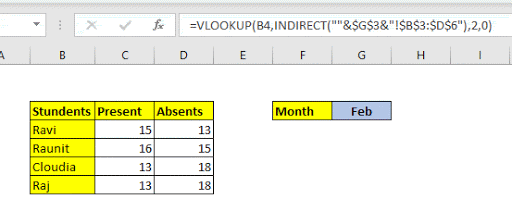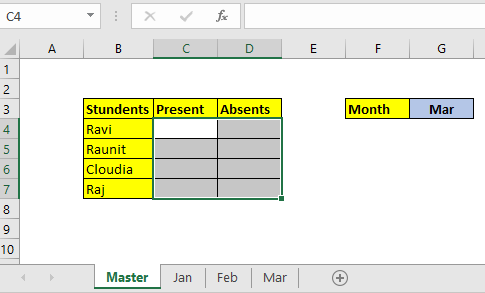How to Lookup From Variable Sheets in ExcelImagine you have multiple identical sheets in a workbook that contains identical tables (like attendance records of each month in a separate sheet). Now you want to create a dashboard that shows you the total attendance for the month. Now having each month's data on the dashboard at the same time is not a good option. We want a drop down to select the month. We need a VLOOKUP formula to look at from the chosen month's sheet.

In simple words, we need a lookup formula to look up from variable sheets.

As the gif above shows, we will be using VLOOKUP and INDIRECT function together to look up from multiple sheets, based on their names.

Generic Formula for Looking Up Multiple Sheets

 =VLOOKUP(lookup_value,INDIRECT(""&sheet_name_reference&"!lookup_table"),col_index,0)

Lookup_value: This is the value you are looking for in the lookup table.

Sheet_name_reference: This is the reference of the cell that contains the name of the sheet.

Lookup_table: This is the table reference in which you want to lookup for lookup_value. It can be a named range, table or absolute reference. It should be the same in all the sheets.

Col_Index: This is the column number in the table from which you want to retrieve value. If you are familiar with VLOOKUP Function, you know what it is.

So let's hop on an example.

Example: Retrieve Attendance for Selected Month

So we have a workbook that maintains the attendance of my Excel students. Each month's data is saved separately in different sheets. The name of the sheet is set to the name of months. For now I have three months data, but there will be more of course.

I want to create a report that shows the attendance of the selected month. The month can be selected from a drop down list. The formula should be able to look up from that sheet automatically, even if a new sheet is  added to it.So, we prepare the above table. The Cell G3 we created a drop down list using a drop down list.

The lookup value is in B4. The sheet_name_reference is in G3. The lookup table in all the sheets is B3:AZ100. We would like to retrieve value from 2 columns. So we write this formula in C4 and drag it down. Similarly, for absent values we change the column index.

 =VLOOKUP(B4,INDIRECT(""&\$G\$3&"!\$B\$3:\$Az\$100"),2,0)How does it work?

The formula is solved inside out. First let's see how it is solved step by step.

Assuming that G3 contains Jan.

 =VLOOKUP(B4,INDIRECT(""&\$G\$3&"!\$B\$3:\$Az\$100"),2,0) =VLOOKUP(B4,INDIRECT("Jan!\$B\$3:\$Az\$100"),2,0) =VLOOKUP(B4,Jan!\$B\$3:\$Az\$100,2,0) =7

So, first the statement ""&\$G\$3&"!\$B\$3:\$Az\$100" is resolved to a string "Jan!\$B\$3:\$Az\$100".  Next the INDIRECT function converts this string into actual reference. And finally we got the formula VLOOKUP(B4,Jan!\$B\$3:\$Az\$100,2,0). And this finally resolves to 7. Now if you change the sheet name in the G3 the value reference text will be changed. And this is how you look up from variable sheets in Excel.

I hope this was helpful to you. If you have any questions or have a different lookup to carry out, let me know in the comments section below. I will be happy to help you. Till then keep Excelling.

Related Articles:

Use VLOOKUP from Two or More Lookup Tables | To lookup from multiple tables we can take an IFERROR approach. Looking up from multiple tables takes the error as a switch for the next table. Another method can be an If approach.

How to do Case Sensitive Lookup in Excel | the excel's VLOOKUP function isn’t case sensitive and it will return the first matched value from the list. INDEX-MATCH is no exception but it can be modified to make it case sensitive. Let’s see how…

Lookup Frequently Appearing Text with Criteria in Excel | The lookup most frequently appears in text in a range we use the INDEX-MATCH with MODE function. Here's the method.

Popular Articles:

50 Excel Shortcuts to Increase Your Productivity | Get faster at your task. These 50 shortcuts will make you work even faster on Excel.

How to use Excel VLOOKUP Function| This is one of the most used and popular functions of excel that is used to look up value from different ranges and sheets.

How to use the Excel COUNTIF Function| Count values with conditions using this amazing function. You don't need to filter your data to count specific values. Countif function is essential to prepare your dashboard.

How to Use SUMIF Function in Excel | This is another dashboard essential function. This helps you sum up values on specific conditions.

Terms and Conditions of use

The applications/code on this site are distributed as is and without warranties or liability. In no event shall the owner of the copyrights, or the authors of the applications/code be liable for any loss of profit, any problems or any damage resulting from the use or evaluation of the applications/code.